Next: 6.4 Associated Flags and Up: 6.3 Description of the Previous: 6.3.1 Determination of the

6.3.2 Determination of the Flux Values

In contrast to the original IUESIPS PHOTOM, the raw DN values are converted to linearized (i.e., photometrically corrected) FN values directly from the effective exposure time associated with each level of the ITF. For a given pixel the FN associated with level i of the ITF is:

FNi = T(i)

where T(i) is the effective exposure time in seconds for level i.

There are four basic methods for the determination of the FN of a raw science image pixel from a single ITF pixel (for the case where the displacement coordinates of the science image are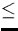0.125 pixel from the ITF pixel coordinate). In the following discussion, let DN(ITF1) represent the DN level of the relevant pixel in the first ITF level; let DN(ITF12) represent the DN level of the relevant pixel in the twelfth ITF level; let DNraw represent the DN level of the science image pixel; and let DNsat represent the DN level of saturation for the relevant pixel. Then the four alternatives are:

• Fully calibrated data: When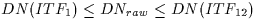, the FN is determined with a linear interpolation algorithm. The interpolation is performed between the two ITF levels that bound the input DN in intensity.
• Saturated data: When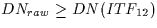and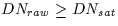, the pixel is considered to be saturated and the FN is set to a constant (the FN value for the top level of the ITF; see Table 6.1). DNsat has been determined on a pixel-by-pixel basis through analysis of the individual ITF curves and is defined to be the DN at which the slope of the ITF curve (DN versus exposure level) approaches zero. Since ITF curves vary considerably from pixel to pixel, the DN level corresponding to saturation varies from pixel to pixel. The flagging of saturated data is broken down into two separate cases; in either case, the FN for the pixel in question is set to the FN of the top level of the ITF:
Case 1:
DNraw = DN(ITF12). These pixels are flagged with the saturation flag of -1024.
Case 2:
DNraw > DN(ITF12). These pixels are flagged with both the saturation flag of -1024 and a positive extrapolation flag of -256. In this instance, the assignment of an extrapolation flag serves only to identify the fact that the DN exceeds that of the top level of the ITF; no extrapolation of the FN data is performed.
• Positively extrapolated data: When DNraw > DN(ITF12) and DNraw < DNsat, the FN is computed by a two-point extrapolation from the top two levels of the ITF. These pixels are flagged with the positive extrapolation flag of -256.
• Negatively extrapolated data: When DNraw < DN(ITF1), the FN is computed with a two-point extrapolation from the bottom two levels of the ITF. However, the pixel is only flagged with an indication of negative extrapolation if the extrapolation is considered excessive. For this purpose, a negative extrapolation reference image'' has been created by determining the 50% intensity level of the ITF null image, and smoothing these data with a 5-point boxcar in two-dimensions. Consequently, negative extrapolations are only considered excessive and flagged if DNraw < DNnegextrefimage. Excessive negative extrapolations are flagged with a value of -128.

When there is significant misalignment (>0.125 pixel) between the raw science image and the ITF, a 4 × 4 matrix of ITF pixels surrounding the location in question is used to compute the relevant FN. Using the raw science image DN, an FN value is computed at each of the 4 × 4 locations in the matrix, using the above described single-pixel scenarios. The median FN in the matrix is computed, and then deviant values are eliminated from the FN matrix and replaced with the median FN. FN values are considered to be deviant if they are different from the median by more than 100 FN. Once the FN matrix has been defined and deviant values replaced, the matrix is fit with a spline surface which is evaluated at the final coordinate location desired for the final FN value.

The FNs determined during the PHOTOM procedure have values which range between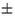1024. Any FN values originally exceeding these limits are clipped and set to the respective limit value.Next: 6.4 Associated Flags and Up: 6.3 Description of the Previous: 6.3.1 Determination of the
Karen Levay
12/4/1997